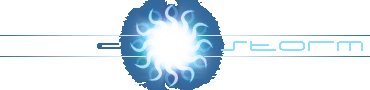::Add RageStorm to Favorites!::Tutorial - Text Blitting
Author:Arkon
Category:DOS Graphics
Views:7818
Blitting text is a really simple thing.
DOS has done most of the job for us, and it's called ROM FONT.
Altough I heard that there are some ways to change this font but it's not the issue.
The ROM FONT may be found at 0xF000FA6EL(Hex).
The ASCII structure is easy:
Every ASCII character is a bitmap of 8X8(Pixels) and it's built of 8 bytes and each bit in the bytes represents a pixel.
So 8(bytes)*8(bits)=64 which gives us 8X8(pixels)font.
Now, to reach a bitmap of a desired character we multiple the character's ASCII value by 8(and add it to the memory location of the font).
Ok, so now you might wonder how come that from 8 BYTES we get a character 'cause unlike the sprite method 8 BYTES is 8 pixels,
so think that instead of BYTE=PIXEL(sprite method), we have a BIT in the BYTE=PIXEL.
So our drawing function will calculate the starting position of the character we look for and read BIT by BIT.

Note: Bit just has two options(0 or 1) so in the drawing function 1 means to draw a pixel(in any color we like) and 0 means to skip.

Take a look how the letter 'H' looks(In mode 13h) in the memory, the red lines seperate the BYTES.And this is how it looks on the Screen as a BITMAP:Character Drawing Function
 ```#define CHARW 8 #define CHARH 8 unsigned char color = 15; void drawchar(int x, int y, char c) { char *work_byte; unsigned char work_bit = 128; work_byte = rom_font +(c << 3);//Character poistion in memory for (int iy = 0; iy < CHARH; iy++) { work_bit = 0x80;//Init the working bit, 'cause if we we are here, it means we start a new byte. for (int ix = 0; ix < CHARW; ix++) { if (*work_byte & work_bit) put_pixel(x +ix, y +iy, color); work_bit >>= 1;//Going to the next bit(/pixel) } work_byte++;//Going to the next Byte(/line) } } ```

Ok well you are confused from the bits, huh??
Well it's easy, but before I start, remember one important thing for the drawing function:BINARY SYSTEM is the basis for BITS!!!!(duh)

work_bit is 128, which is the last bit which is the first pixel. :)
work_byte is the starting position of the font plus character's value multipled by 8(As i said above).
if (*work_byte & work_bit) - here, we check whether the bit is 1 or 0(If the result is 1, we draw the pixel)
We read the pointer of work_byte 'cause we want the byte it points on, not the number of the byte it points.
And then we ADD(LOGIC) the work_bit to the work_byte, so we actually check whether the bit(work_bit) is 1 in the byte.
work_bit >>= 1 is the same as work_bit /= 2 - We do this 'cause we want to scan every bit(128, 64, 32, 16, 8, 4, 2, 1 - 8 bits).
work_byte++ - We get the next byte(next line of the bitmap).

Note: if you have no idea about BITS, visit here.
If you still don't understand it, you are a dumb(j/k) read the code a few more times and it will be clearer,
or I'm a bad teacher :(

 ```void writestring(int x, int y, char *string) { for (int index = 0; string[index] != 0; index++) drawchar(x +(index << 3), y, string[index]); // We multpile the character position on the string by 8 in order to get it's position on the screen. } void writestring(int x, int y, int number) {//Same function, but here we just convert the number into a string and blits it. char string; itoa(number, string, 10); for (int index = 0; string[index] != 0; index++) drawchar(x +(index << 3), y, string[index]); } ```

Source Code:
DJGPP - text.c
Turbo C - text.cpp

NOTE:
Comments that will hurt anyone in any way will be deleted.